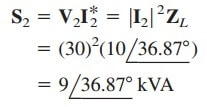# Easy Ideal Autotransformer CalculationsUnlike the conventional two-winding transformer we have considered so far, an autotransformer has a single continuous winding with a connection point called a tap between the primary and secondary sides. As a start, we will learn about the ideal autotransformer.

Contents

The tap is often adjustable so as to provide the desired turns ratio for stepping up or stepping down the voltage. This way, a variable voltage is provided to the load connected to the autotransformer.

An autotransformer is a transformer in which both the primary and the secondary are in a single winding.

## Ideal Autotransformer

Figure.(1) shows a typical autotransformer.

As shown in Figure.(2), the autotransformer can operate in the step-down or step-up mode. The autotransformer is a type of power transformer. Its major advantage over the two-winding transformer is its ability to transfer larger apparent power.

Another advantage is that an autotransformer is smaller and lighter than an equivalent two-winding transformer.

However, since both the primary and secondary windings are one winding, electrical isolation (no direct electrical connection) is lost.

The lack of electrical isolation between the primary and secondary windings is a major disadvantage of the autotransformer.

Some of the formulas we derived for ideal transformers apply to ideal autotransformers as well.

For the step-down autotransformer circuit of Figure.(2a), we use the equation

As an ideal autotransformer, there are no losses, so the complex power remains the same in the primary and secondary windings:

Equation.(2) can also be expressed with rms values as

Thus, the current relationship is

For the step-up autotransformer circuit of Figire.(2b),

The complex power given by Equation.(2) also applies to the step-up autotransformer so that Equation.(3) again applies. Hence, the current relationship is

A major difference between conventional transformers and autotransformers is that the primary and secondary sides of the autotransformer are not only coupled magnetically but also coupled conductively. The autotransformer can be used in place of a conventional transformer when electrical isolation is not required.

## Ideal Transformer Examples

Let us review the ideal transformer examples below:

### Ideal Transformer Example 1

Compare the power ratings of the two-winding transformer in Figure.(3a) and the autotransformer in Figure.(3b).Solution:
Although the primary and secondary windings of the autotransformer are together as a continuous winding, they are separated in Figure.(3b) for clarity.

We note that the current and voltage of each winding of the autotransformer in Figure.(3b) are the same as those for the two-winding transformer in Figure.(3a). This is the basis of comparing their power ratings.

For the two-winding transformer, the power rating isFor the autotransformer, the power rating iswhich is 21 times the power rating of the two-winding transformer.

### Ideal Transformer Example 2

Refer to the autotransformer circuit in Figure.(4). Calculate: (a) I1, I2, and Io if ZL = 8 + j6 Ω, and (b) the complex power supplied to the load.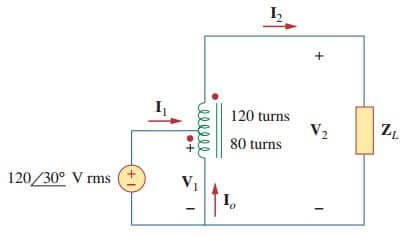Solution:
(a) This is a step-up autotransformer with N1 = 80, N2 = 120, V1 = 120 ∠30◦, so Equation.(5) can be used to find V2 byor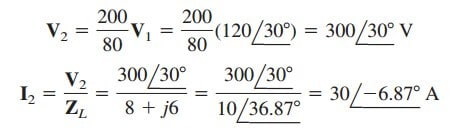ButorAt the tap, KCL gives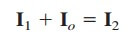or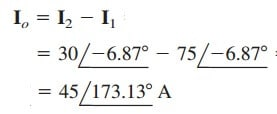(b) The complex power supplied to the load is